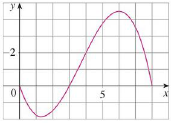Chapter 5.2, Problem 51E### Single Variable Calculus: Early Tr...

8th Edition
James Stewart
ISBN: 9781305270343

#### Solutions

Chapter
Section### Single Variable Calculus: Early Tr...

8th Edition
James Stewart
ISBN: 9781305270343
Textbook Problem

# For the function f whose graph is shown, list the following quantities in increasing order, from smallest to largest, and explain your reasoning.(A) ∫ 0 8 f ( x ) d x (B) ∫ 0 3 f ( x ) d x (C) ∫ 3 8 f ( x ) d x (D) ∫ 4 8 f ( x ) d x (E) f′(1)To determine

To list: The following integral in the increasing order.

(A) 08f(x)dx

(B) 03f(x)dx

(C) 38f(x)dx

(D) 48f(x)dx

(E) f(1)

Explanation

Given:

The graph of the function f is shown in Figure 1.

Calculation:

Plot the graph of the function f as shown below:

Refer the figure 1.

Consider the area under the graph are A1, A2, and A3.

The area A1 is negative as it is below the x-axis.

The area A1 is equal to integral 03f(x)dx.

The area A2 is equal to integral 34f(x)dx.

The area A3 is equal to integral 48f(x)dx.

The first smallest value among the integral is 03f(x)dx.

The area denoted by 03f(x)dx is the maximum negative area under the function f.

The second smallest area under the plot is f(1).

The value of f(1) is the value of the tangent at x=1.

The value of f(1) will be between 0 and 1.

The third smallest area under the plot is 08f(x)dx.

This is the difference of maximum positive area and maximum negative area.

The largest area under the plot is 38f(x)dx.

The area denoted by 38f(x)dx is the maximum negative area under the function f.

The second largest area under the plot is 34f(x)dx

### Still sussing out bartleby?

Check out a sample textbook solution.

See a sample solution

#### The Solution to Your Study Problems

Bartleby provides explanations to thousands of textbook problems written by our experts, many with advanced degrees!

Get Started